# ILP Part 2 – Multiplication

This is the second part of the ILP series. For your convenience you can find other parts in the table of contents in Part 1 – Boolean algebra

Previously we defined Boolean algebra operators using ILP. Today we are going to implement multiplication using the tools we defined last time.

# Basics

In ILP we are not able to multiply variables because we need to stick to linear constraints. It is also not clear how can we utilize only addition to calculate a multiplication. However, by reducing the size of domain we will be able to do that.

## Decomposition

First, we need to use some hack: we need to limit the maximum possible value of a variable. Let’s assume that we want to do the following: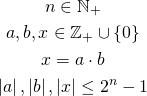So we say that our variables are not greater than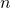-th power of two. For now we also stick to non-negative values, we will generalize the operator later.
Now we are able to decompose value and retrieve digits: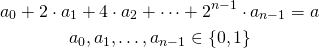We basically represent the variable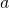in binary notation. Every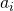is a binary variable for consecutive power of two. This representation is well defined for every non-negative number so there is no ambiguity here.

## Reduced size – problem?

Representing the variable in binary notation looks like a great hack. Indeed, we have just thrown out infinite number of possible values. But in fact it is not a problem. We need to remember that we are going to solve the problem using solver which will probably perform operations using double values which are already limited. We are only introducing a bit lower limit but in real world it doesn’t bother us. It is not likely that we will need to perform calculations on infinite values.

## Long multiplication

Now we are able to perform multiplication. We are going to do that the same way as we were taught at school (you remember that, don’t you?). We will multiply every single pair of digits and sum the results. First let’s define variables and decomposition: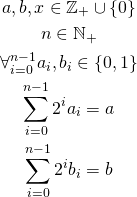We also introduce the following notation: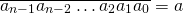The line means that the variables are particular digits and should be read as a whole.
No let’s present the multiplication formula: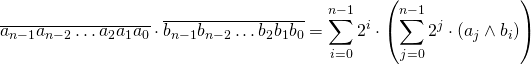It looks a bit odd, however, it is in fact really easy. We are taking every pair of digits and multiplying them using conjunction (defined in previous part), we also handle powers of two. Let’s see an example: we multiply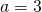and, each treated as a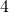bit number (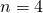).
First let’s decompose the values: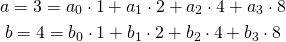We get: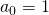,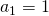,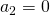,,,,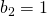,. Now we perform the long multiplication: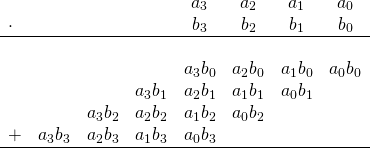Using the previously found values we obtain:The result is equal to, which comes from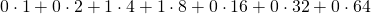. We could write our result using the following expression: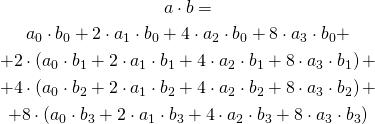Which in our example is equal to: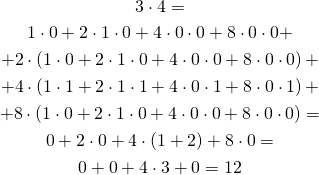Since we cannot multiply two variables (because we would get a non-linear constraint), we need to represent every multiplication of two digits (two binary variables) as their conjunction.

# Performance

As we can see, presented approach is rather slow. Imagine that we are going to multiply two integers with 30 digits each. This requires over 900 temporary variables (we need to calculate conjunction for every pair of integers). In fact we need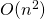temporary variables to perform multiplication. When we come to calculatingfunction we will see that we can do better using only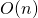variables.

# Summary

To sum up, our algorithm comes as follows: we first find the unsigned magnitude representations of multiplicand and the multiplier, next we calculate conjunction of every digit of the multiplicand and every digit of the multiplier, and finally we form the result by summing the digits multiplied by correct weights.

In the next part we are going to define other common operators: division, remainder. We will also calculate powers and roots.